﻿ 20-sim webhelp > Library > Iconic Diagrams > Mechanical > Rotation > Components > clutch

# clutch

Iconic Diagrams\Mechanical\Rotation\Components

C

V

CV

SCVS

LuGre

## Use

Domains: Continuous. Size: 1-D. Kind: Iconic Diagrams (Rotation).

## Introduction

The clutch models model represent friction relative to other objects. The amount of friction depends on the normal force that is applied and the friction function that is used. The normal force is given by the input signal FN.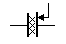The port p of the clutch model has separate high and low terminals. The equations are:

p.T = p_high.T = p_low.T

p.omega = p_high.omega - p_low.omega

Due to the use of normal force, the clutch models all have a fixed torque out causality. The constitutive equations are therefore described as:

p.T = Fn * f(p.omega);

with f the friction function.

## Description - C

This model represents bearing with friction force described as coulomb friction. The amount of friction depends on the normal force that is applied and the friction function that is used:

p.T = Tc*tanh(slope*p.omega);

Tc: coulomb friction

slope: the steepness of the coulomb friction curve.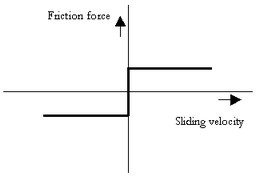## Interface - C

 Ports Description p Both terminals of port p (Rotation). Causality Fixed torque out Input Fn Normal force [N] Parameters Tc slope Coulomb friction [N.m] Steepness of Coulomb friction curve [s/rad]

## Description - V

This model represents a clutch with friction force described as viscous friction. The amount of friction depends on the normal force that is applied and the friction function that is used:

p.T = Fn*mu_v*p.omega;

Fn: normal force (given by the input signal Fn)

mu_v: the viscous friction coefficient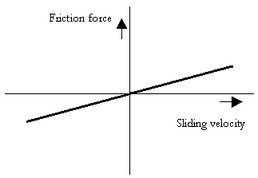## Interface - V

 Ports Description p_high, p_low Both terminals of port p (Rotation). Causality Fixed torque out Input Fn Normal force [N] Parameters mu_v Viscous friction coefficient [m.s/rad]

## Description - CV

This model represents a clutch with friction force described as coulomb plus viscous friction. The amount of friction depends on the normal force that is applied and the friction function that is used:

p.T = Fn*(Tc*tanh(slope*p.omega) + mu_v*p.omega);

Fn: normal force (given by the input signal Fn)

mu_v: the viscous friction coefficient

Tc: the coulomb friction coefficient

slope: the steepness of the coulomb friction curve.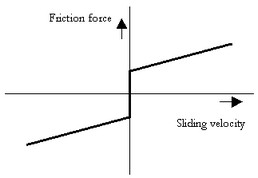## Interface - CV

 Ports Description p_high, p_low Both terminals of port p (Rotation). Causality Fixed torque out Input Fn Normal force [N] Parameters mu_v Tc slope Viscous friction coefficient [m.s/rad] Coulomb friction [N.m] Steepness of Coulomb friction curve [s/rad]

## Description - SCVS

This model represents a clutch with friction force described as static plus coulomb plus viscous plus Stribeck friction. The amount of friction depends on the normal force that is applied and the friction function that is used:

p.T = Fn *

(( Tc + (mu_st*abs(tanh( slope*p.omega )) - Tc)

* exp( -((p.omega / v_st)^2 )) ) * sign(p.omega)

+ mu_v * p.omega);

Fn: normal force (given by the input signal Fn)

mu_s: the static friction coefficient

mu_v: the viscous friction coefficient

Tc: the coulomb friction coefficient

slope: the steepness of the coulomb and static friction curve.

v_st: the characteristic Stribeck velocity.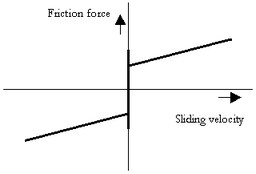## Interface - SCVS

 Ports Description p_high, p_low Both terminals of port p (Rotation). Causality Fixed torque out Input Fn Normal force [N] Parameters mu_s mu_v Tc slope v_st Static friction coefficient [m] Viscous friction coefficient [m.s/rad] Coulomb friction [N.m] Steepness of Coulomb friction curve [s/rad] Characteristic Stribeck velocity [rad/s]

## Description - LuGre

This model represents a clutch with friction force described by the LuGre friction model. The amount of friction depends on the normal force that is applied and the friction function that is used:

p.T = FN*f_lg(p.omega);

Fn: normal force (given by the input signal Fn)

f_lg: the LuGre friction model

## Interface- LuGre

Ports

Description

p_high, p_low

Both terminals of port p (Rotation).

Causality

Fixed torque out

Input

Fn

Normal force [N]

## Parameters

Tc

mu_s

mu_v

v_st

mu_k

Coulomb friction coefficient

Static friction coefficient [m]